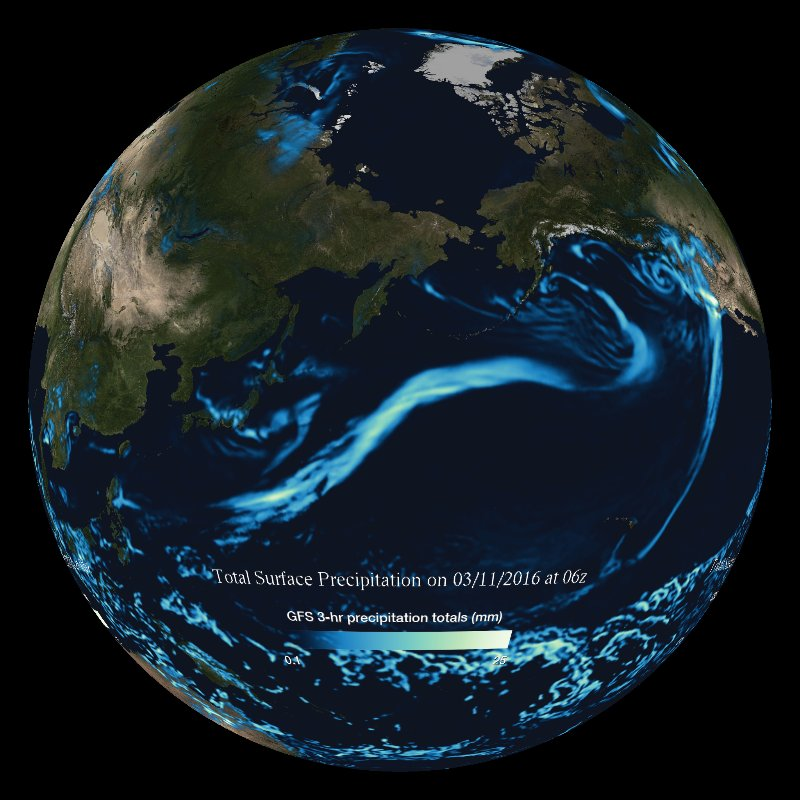# GFS Forecast Model: Precipitation - Real-timeDescription

Meteorologists use many tools to predict the weather. They use past data such as temperature observations, real-time data such as radar and satellite images, and models that look into the future. Many different parameters are plotted using the numerical forecast models, which are generated using computers. The models consist of numerical equations which use current conditions as the inputs. The resulting outputs are forecasts for what is likely to happen in the future, based on those initial conditions. There are many different models that all attempt to do the same thing. The Global Forecast System model is used in this visualization.

Predicted amounts of precipitation are shown in this imagery from the NOAA Global Forecast System weather model. Each time step is an accumulation of the predicted precipitation amounts over a 3-hour period. Light blue areas are low precipitation amounts; bright green/white areas are heavy amounts. The amount of precipitation is shown as the accumulated amount over the three hours in between each time increment of the model. The values are displayed in inches or mm of liquid water (rain), though depending on the temperatures, it may precipitate as snow. In those cases a general rule of thumb is that 1 inch of liquid water equals 10 inches of snow. The model updates every six hours and provides forecasts for 10 days (240 hours) out from the start time.

Related Datasets